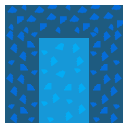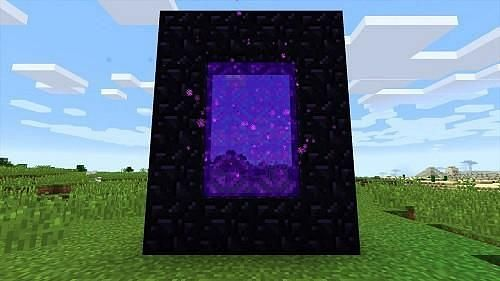Uh Oh! It seems you’re using an Ad blocker!

Since we’ve struggled a lot to makes online calculations for you, we are appealing to you to grant us by disabling the Ad blocker for this domain.# Nether Portal Calculator

Calculate:

X

Y

Z## 3D Distance Calculator

X1

X2

Y1

Y2

Z1

Z2

Table of Content

 1 What is Nether? 2 Nether Portal: 3 Construction of A Nether Portal: 4 How To Design A Nether Portal? 5 Can nether portal be designed as large as you want? 6 What is the maximum size of an ender portal? 7 Can you take animals to the nether portal?

Get the Widget!

Add this calculator to your site and lets users to perform easy calculations.

Feedback

How easy was it to use our calculator? Did you face any problem, tell us!

An online nether calculator helps you to design a nether portal in between a nether and the overworld with the help of the coordinates. Let us have a look of this hypothetical portal design in minecraft gaming. Move on!

## What is Nether?

“A drastic hell-like structure that is filled with the lava or fire is known as nether”.

### Nether Portal:

Sometimes in minecraft gaming, players have to move to a destination that is too far from their starting point. That is why it is a preferable practice to build up a nether portal that helps you take to the destination relatively faster than the normal world (Overworld). An online nether portal calculator is the best method to build up a proper nether portal.Travelling from overworld to nether is an easy process. But before that, you need to understand how to access a nether portal.### Construction of A Nether Portal:

When a player wants to rush to the nether, he/she must be capable of building a nether portal. First, you must build a rectangular frame which is known as an obsidian frame. After you build it, create a portal inside this frame to have a proper approach to the nether. Once you make the portal, activate this portal by putting fire charge, lava or anything that could ignite the portal within the obsidian frame.

#### Nether Coordinates:

The nether to overworld ratio is 1:8 in terms of the distance. It means that covering a distance of one block in nether is equivalent to that of covering a distance upto eight blocks in the overworld. To convert minecraft overworld to nether coordinates, we need to follow these conversions.

For X coordinate:

Divide the coordinate by a factor of 8.

X = floor(overworld x coordinate / 8)

For Y coordinate:

it will remain the same.

For Z coordinate:

Divide by a factor of 8.

Z = floor(overworld z coordinate / 8)

Also, a minecraft nether portal calculator determines the coordinates of nether even more accurately than manual calculations.

#### Overworld Coordinates:

If you seek for converting nether coordinates to overworld, you need to assume the following basic formulas:

For X coordinate:

Multiply the x coordinate of the nether portal by a factor of 8.

X = floor(nether x coordinate * 8)

For Y coordinate:

It will again remain the same.

For Z coordinate:

Multiply this coordinate by a factory of 8 also.

Z coordinate = floor(nether z coordinate * 8)

Note: the term floor means that if your results are in decimal numbers, they will be approximated to a nearby absolute number. The fact behind this mystery is that you can never get exact coordinates every single time because it’s a hypothetical approach.

#### Minecraft Distance:

It is also a significant practice to find the distance between overworld and nether. You need to follow the basic 3D distance formula to determine the gap with the help of the coordinates. Our free online minecraft distance calculator determines the distance between normal world and nether portal accurately. Anyhow, the distance formula is as follows:

$$3D Distance = \sqrt{ {\left(x_{2} – x_{1}\right)}^2 + {\left(y_{2} – y_{1}\right)}^2 + {\left(z_{2} – z_{1}\right)}^2}$$

### How To Design A Nether Portal?

It is a tricky approach to construct a nether portal but not impossible at all. You have to work for considerable coordinates for nether and overworld so as to depict the final design. Moreover, you must know how to link nether portals so that more gateways may be formed for you during the game towards the destination. What about solving a few examples to clarify the idea!

Example # 01:

Just suppose you, being a player, want to go to a village that is very far from where you are.
The coordinates corresponding to your current position are as follows:

X = 65, Y = 80, Z = 96.

Determine the coordinates of nether in order to design a nether portal so to minimize the duration of your journey to the village. Also find the distance between nether and overworld coordinates.

Solution:

For overworld to nether coordinates, we have:

X = floor(overworld x coordinate / 8)

X = floor(65 / 8)

X = 8

The Y coordinate will remain the same by default.

Y = 80

Determining z coordinate as follows:

Z = floor(overworld z coordinate / 8)

Z = floor(96 / 8)

Z = 12

For better results, you can use our free online minecraft nether coordinate calculator.

Now, determining the distance as follows:

$$3D Distance = \sqrt{ {\left(x_{2} – x_{1}\right)}^2 + {\left(y_{2} – y_{1}\right)}^2 + {\left(z_{2} – z_{1}\right)}^2}$$

$$3D Distance = \sqrt{ {\left(65 – 8\right)}^2 + {\left(80- 80\right)}^2 + {\left(96 – 12\right)}^2}$$

$$3D Distance = \sqrt{ {\left(57\right)}^2 + {\left(0\right)}^2 + {\left(84\right)}^2}$$

$$3D Distance = \sqrt{ 3249 + 0 + 7056}$$

$$3D Distance = 101.513$$

Here, our free online nether calculator finds the distance more accurately to nullify the error.

Example # 02:

You are given the nether coordinates as:

X = 100, Y = 300 Z = 600

Determine the overworld coordinates:

Solution:

Converting nether coordinates to overworld as follows:

X = floor(nether x coordinate * 8)

X = floor(100 * 8)

X = 800

The Y coordinate remains the same.

Y = 300

For third coordinate, we have:

Z = floor(600 * 8)

Z = 4800

### How Nether Calculator Works?

You will get many calculators in front of you for determining the parameters required to design a nether portal. But the free minecraft nether portal calculator is the best among all. Let us guide you to the proper use of our calculator.

Input:

• First of all, select either “nether coordinates” or “overworld coordinates” from the drop down menu.
• Enter the x, y and z coordinates in the boxes.
• Click ‘calculate’

Now, if you wish to determine the 3d distance as well:

• Enter coordinates of both nether and overworld.
• Hit the calculate button.

Output:

The minecraft portal calculator calculates:

• Either “nether coordinates” or “overworld coordinates”
• The distance between nether and overworld depending upon the coordinates of both worlds.

## FAQ’s:

### Can nether portal be designed as large as you want?

No, the dimensions for a nether portal include a minimum of 4 * 5 to a maximum of 23 * 23. Beyond this range, you remain incapable of building a suitable nether portal. in case of any confusion, subject to our free online nether calculator for free.

### What is the maximum size of an ender portal?

The ender portal must have the dimensions of 3 * 3 blocks. Also, its direction should be towards the center.

### Can you take animals to the nether portal?

Yes, but you can only bring cows, pigs and sheeps through the portal. However, there is not much use of these animals but when you face a very shortage of everything, then you could use them in your nether farm. Also you can bring dogs to your nether farm.

## Conclusion:

A nether portal allows you to cover a large distance in a much shorter span of time than overworld. The nether portal calculator is broadly used in the galaxy of minecraft gaming where it becomes necessary for a player to cover huge distances without being hurt by any enemy and dangerous animals. Without building a nether portal, you would not be able to reach your destination ever.

From the source of minecraft: Portal Connections, Calculating Portal Positions, Chunk Loading.